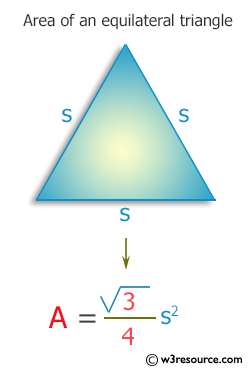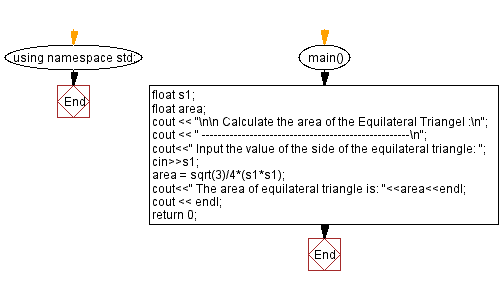﻿ C++ Exercises: Calculate area of an equilateral triangle - w3resource

# C++ Exercises: Calculate area of an equilateral triangle

## C++ Basic: Exercise-53 with Solution

Write a C++ program to calculate the area of an equilateral triangle.

Visual Presentation:Sample Solution:

C++ Code :

``````#include<iostream> // Including input-output stream header file
using namespace std; // Using the standard namespace
#include<math.h> // Including the math library header file for mathematical operations

int main() { // Start of the main function

float s1; // Declaring a variable to store the side length of the equilateral triangle
float area; // Declaring a variable to store the area of the equilateral triangle

cout << "\n\n Calculate the area of the Equilateral Triangle :\n"; // Displaying the purpose of the program
cout << " ----------------------------------------------------\n";

cout << " Input the value of the side of the equilateral triangle: "; // Prompting the user to input the side length
cin >> s1; // Taking input of the side length from the user

area = sqrt(3) / 4 * (s1 * s1); // Calculating the area of the equilateral triangle using the formula
cout << " The area of the equilateral triangle is: " << area << endl; // Displaying the calculated area

cout << endl; // Displaying an empty line for better readability
return 0; // Returning 0 to indicate successful program execution
}
``````

Sample Output:

```Calculate the area of the Equilateral Triangle :
----------------------------------------------------
Input the value of the side of the equilateral triangle: 5
The area of equilateral triangle is: 10.8253
```

Flowchart:C++ Code Editor:

What is the difficulty level of this exercise?

﻿